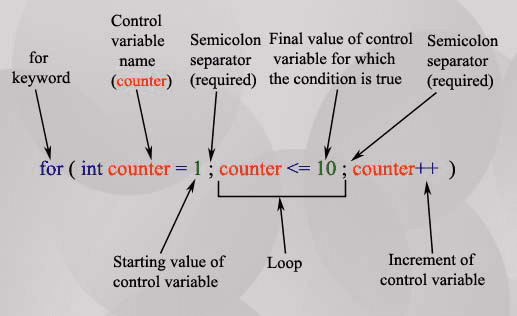# C for loop

## Description

A "For" Loop is used to repeat a specific block of code (statements) a known number of times. The for-loop statement is a very specialized while loop, which increases the readability of a program. Here is the syntax of the of for loop.

for ( initialize counter ; test counter ; increment counter)
{
execute the statement(s);
}
• initialize counter : Initialize the loop counter value.
• test counter : Verify the loop counter whether the condition is true.
• increment counter : Increasing the loop counter value.
• execute the statement : Execute C statements.

Note : The for-loop must have two semi-colons between the opening and closing parenthesis.

The following picture has clearly described the for loop syntax.## Why For Loops?

1. " For" loops execute blocks of code over and over again.

2. It is clear to a developer exactly how many times the loop will execute before the loop starts.

3. The Syntax of the for loop is almost same to other programming languages.

## For loop repetition statement

Here are some example of for loop repetition statements.

The following code prints the numbers from 1 to 100 in increments of 1.

```for ( int x = 1; x <= 100 ; x++ )
{
printf("%d\n",x);
}```

The following code prints the numbers from 100 to 1 in increments of -1.

``````for(int x = 100 ; x >= 1; x--)
{
printf("%d\n",x);
}``````

The following code prints the numbers from 8 to 88 in steps of 8

``````for(int x = 8; x <= 88 ; x += 8)
{
printf("%d\n",x);
}``````

The following code prints : 2, 7, 12, 17, 22, 27

``````for(int x = 2; x <= 30 ; x += 5)
{
printf("%d\n",x);
}``````

The following code prints: 66, 60, 54, 48, 42, 36, 30, 24, 18, 12, 6, 0

``````for(int x = 66 ; x >= 0; x -= 6 )
{
printf("%d\n",x);
}``````

## For loop Examples

Example - 1:

The following program calculate the sum of 1+2+3+...+50. The sum is stated in sum = sum + x, where i takes values from 1 to 50.

``````#include<stdio.h>
main()
{
int sum; int x; sum=0;
for(x=1;x<=50;++x)
// x take values in {1,2,3,...,50}
{
sum = sum + x;
}
printf(" 1+2+...+50=%d\n",sum);
}
``````

Output:

``` 1+2+...+50=1275
```

Example - 2:

The following program will ask the user to input 10 integers and find the sum.

``````#include<stdio.h>
main()
{
int z;
int x, sum=0, inpn;
// initialization
for(x=1; x<=10; ++x)
// Ask user 10 times(i.e. x takes 10 integers)
{
printf("Enter #%d: ",x);
scanf("%d",&inpn);
sum = sum + inpn;
// Accumulate the next value
}
printf("Total Sum of 10 numbers = %d\n",sum);
}
``````

Output:

```  Enter #1: 1
Enter #2: 1
Enter #3: 1
Enter #4: 1
Enter #5: 1
Enter #6: 1
Enter #7: 1
Enter #8: 1
Enter #9: 1
Enter #10: 2
Total Sum of 10 numbers = 11  ```

Example - 3:

The following program will ask the user to input 5 numbers and print out the maximum and minimum numbers from the set.

``````#include<stdio.h>
main()
{
int Max, Min, Inpn, x;
printf("Input #1: ");
scanf("%d",&Inpn);
Max = Inpn;
Min = Inpn;
// Call the First number as current maximum and minimum
for(x=2;x<=5;++x)
{
printf("Input #%d: ",x);
scanf("%d",&Inpn);
if(Inpn>Max)
// if the next number is bigger than current maximum, store it
{
Max = Inpn;
}
if(Inpn<Min)
// if the next number is lower than current minimum, store it
{
Min = Inpn;
}
}
printf(" The Maximum # is %d\n",Max);
printf(" The Minimum # is %d\n",Min);
}
``````

Output:

```Input #1: 120
Input #2: 34
Input #3: 0
Input #4: 1234
Input #5: -500
The Maximum # is 1234
The Minimum # is -500
```

Example - 4:

A prime number is a number that is only divisible by 1 and itself. We can check whether a number x is prime by checking if it is divisible by any of the numbers between 2 to x-1. For example if an user input a number say 5, then we will check if 5 is divisible by 2, 3 or 4. If 5 is divisible by 2, 3 or 4 then we can say that 5 is not prime. In the following program we use a for loop to iterate with y over the numbers 2,3,....x-1 and check if y is divided by the number x. Within if statement we use an indicator (z=1) to mark that x is not prime and during the for loop execution if the reminder of x and y is found 0 the break statement will cause the loop to exit.

``````#include<stdio.h>
main()
{
int x, z;
z=0;
printf("Input a number : ",x);
scanf("%d",&x);
for(int y = 2; y<x; y++)
{
if (x%y == 0)
{
// y divides x - not  a prime number
printf("%d is not a prime number",x);
z=1;
break;
}
}
if (z==0)
{
printf("%d is a prime number",x);
}
}
``````

Output:

```Input a number : 7
7 is a prime number```
```Input a number : 8
8 is not a prime number
```

Previous: C if else
Next: C while loop

﻿

```NOT 011100